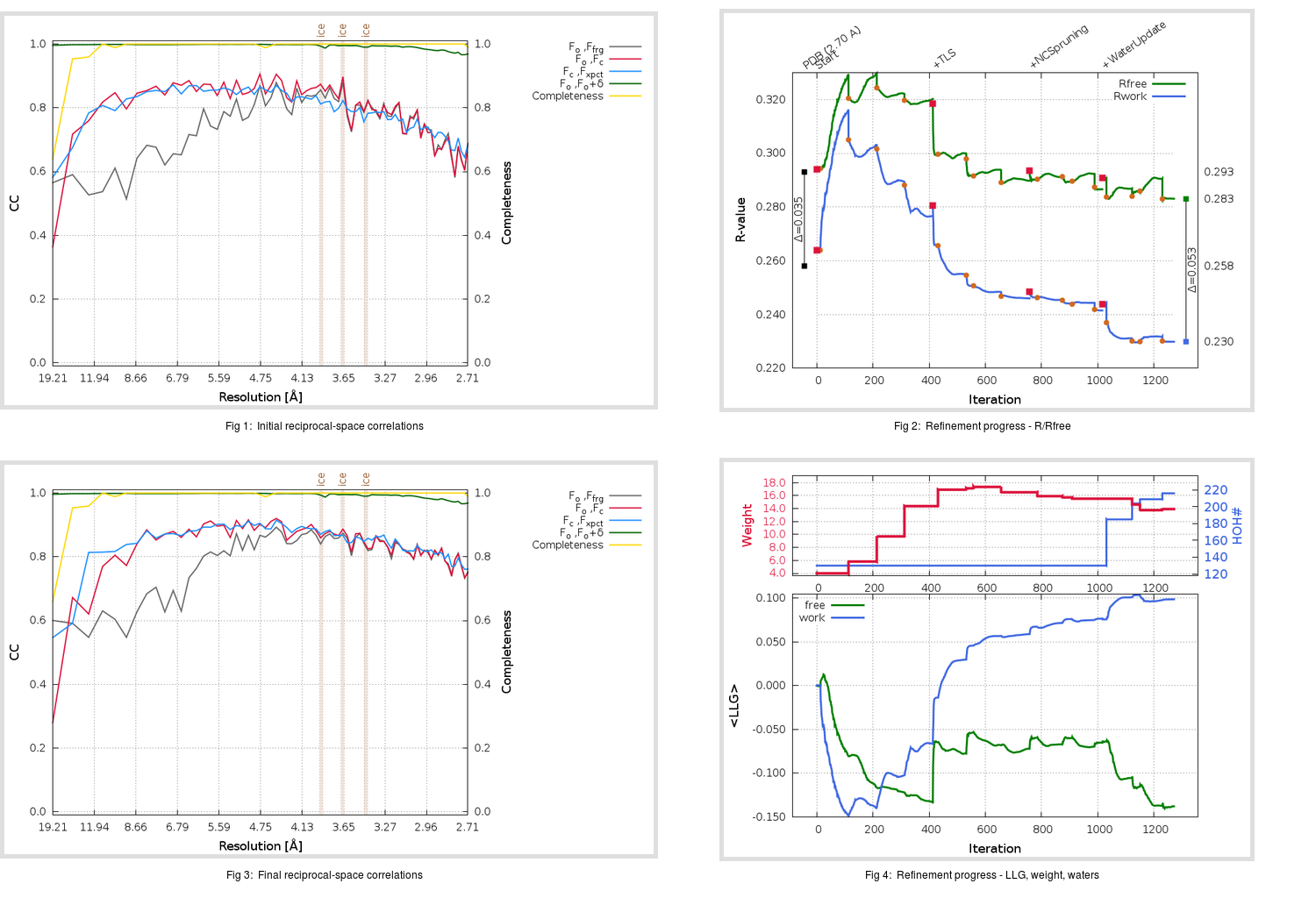Content:

```    Diffraction limits & principal axes of ellipsoid fitted to diffraction cut-off surface:
2.624         1.0000   0.0000   0.0000       a*
2.646         0.0000   1.0000   0.0000       b*
2.650         0.0000   0.0000   1.0000       c*
```

## Deposited

` `
 Date deposited Date data collection Resolution R, Rfree 20200304 20200228 2.70 0.2580 0.2930

Molprobity (CCP4 7.0 version) summary:

```Ramachandran outliers =   0.00 %
favored =  96.48 %
Rotamer outliers      =   0.00 %
C-beta deviations     =     0
Clashscore            =  11.02
RMS(bonds)            =   0.0050
RMS(angles)           =   1.04
MolProbity score      =   1.79
Resolution            =   2.70
R-work                =   0.2580
R-free                =   0.2930
```

```Number of waters      =   130

<B> (all atoms) =   31.59 ( sd =   12.74 ) for       3949 non-hydrogen atoms
<B>   (protein) =   31.85 ( sd =   12.76 ) for       3819 non-hydrogen atoms
<B>     (water) =   24.03 ( sd =    9.51 ) for        130 non-hydrogen atoms
<B>    (others) =    0.00 ( sd =    0.00 ) for          0 non-hydrogen atoms

B min/max       (all non-hydrogen atoms) =    6.30 /   80.12
B min/max   (protein non-hydrogen atoms) =    8.56 /   80.12
B min/max     (water non-hydrogen atoms) =    6.30 /   70.40
B min/max     (other non-hydrogen atoms) =    0.00 /    0.00
```

## BUSTER (re-)refinement

` `

Molprobity (CCP4 7.0 version) summary:

```Ramachandran outliers =   0.83 %
favored =  97.31 %
Rotamer outliers      =   5.56 %
C-beta deviations     =     0
Clashscore            =   2.42
RMS(bonds)            =   0.0116
RMS(angles)           =   1.52
MolProbity score      =   1.72
Resolution            =   2.70
R-work                =   0.2297
R-free                =   0.2830
```

```Number of waters      =   216

<B> (all atoms) =   44.78 ( sd =   21.60 ) for       4035 non-hydrogen atoms
<B>   (protein) =   45.59 ( sd =   21.74 ) for       3819 non-hydrogen atoms
<B>     (water) =   30.33 ( sd =   11.82 ) for        216 non-hydrogen atoms
<B>    (others) =    0.00 ( sd =    0.00 ) for          0 non-hydrogen atoms

B min/max       (all non-hydrogen atoms) =    4.58 /  130.55
B min/max   (protein non-hydrogen atoms) =   11.48 /  130.55
B min/max     (water non-hydrogen atoms) =    4.58 /   79.61
B min/max     (other non-hydrogen atoms) =    0.00 /    0.00
```

Refinement progression:Results:

` `
 File Remark 6M3M_aB_refine.01_04_refine.pdb.gz exact refinement commands are in header 6M3M_aB_refine.01_04_refine.mtz.gz including original deposited data and several re-refinement map coefficients 6M3M_aB_refine.01_04_BUSTER_model.cif.gz including any non-standard compound restraints 6M3M_aB_refine.01_04_BUSTER_refln.cif.gz